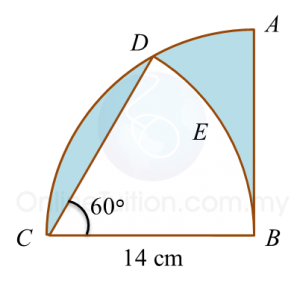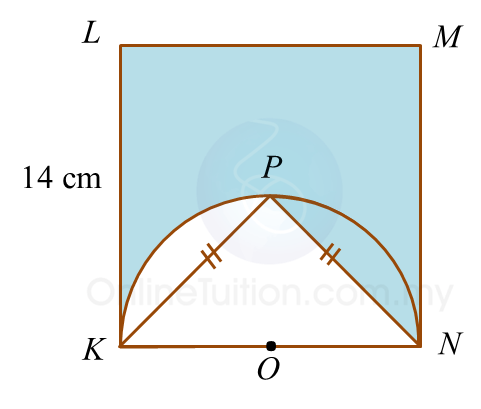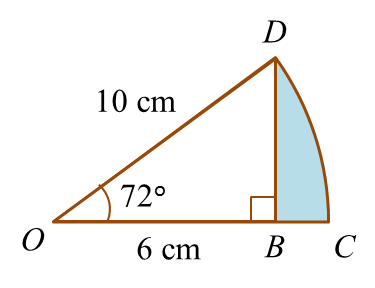# 5.2.2 Circles, PT3 Focus Practice

Question 4:
Diagram below shows two sectors. ABCD is a quadrant and BED is an arc of a circle with centre C.Calculate the area of the shaded region, in cm2.

Solution
:

Question 5:
Diagram below shows a square KLMN. KPN is a semicircle with centre O.Calculate the perimeter, in cm, of the shaded region.

Solution
:

= KL + LM + MN + NP + Arc length PK
= 14 + 14 +14 + 9.90 + 11
= 62.90 cm

    
                 
Question 6:
       In the diagram below, CD is an arc of a circle with centre O.Determine the area of the shaded region.
                                                    
 
 Solution: